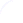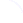Search
 Submission Procedure share: |available in:   PDF (243 kB) PS (389 kB)

get:
 Similar Docs BibTeX Write a comment
get:

DOI:   10.3217/jucs-007-04-0307

### Computational Complexity of the Place/Transition-Net Symmetry Reduction Method

Tommi A. Junttila (Laboratory for Theoretical Computer Science Helsinki University of Technology, Finland)

Abstract:

Computational complexity of the sub­tasks in the symmetry reduction method for Place/Transition-nets is studied. The task of finding the automorphisms (symmetries) of a net is shown to be polynomial time many-one equivalent to the problem of finding the automorphisms of a graph. Deciding whether two markings are symmetric is shown to be a problem equivalent to the graph isomorphism problem. This remains to be the case even if a generator set for the automorphism group of the net is known. The problem of constructing the lexicographically greatest marking symmetric to a given marking (a canonical representative for the marking) is classified to belong to the lower levels of the polynomial hierarchy, namely to be FPNP[log n] - hard but in FPNP. It is also discussed how the self-symmetries of a marking can be exploited. Calculation of such symmetries is classified to be as hard as computing graph automorphism groups. Furthermore, the coverability version of testing marking symmetricity is shown to be an NP-complete problem. It is proven that canonical representative markings and the symmetric coverability problem cannot be combined in a straightforward way.

Keywords: Petri nets, computational complexity, symmetry

Categories: D.2.4, F.2